# Samacheer Kalvi 8th Maths Solutions Term 2 Chapter 4 Information Processing Ex 4.3

Students can Download Maths Chapter 4 Information Processing Ex 4.3 Questions and Answers, Notes Pdf, Samacheer Kalvi 8th Maths Book Solutions Guide Pdf helps you to revise the complete Tamilnadu State Board New Syllabus and score more marks in your examinations.

## Tamilnadu Samacheer Kalvi 8th Maths Solutions Term 2 Chapter 4 Information Processing Ex 4.3

Question 1.

Question (i)
In questions A and B, there are four groups of letters in each set. Three of these sets are like in some way while one is different. Find the one which is different.
A. (a) C R D T (b) A P B Q (c) E U F V (d) G W H X
B. (a) H K N Q (b) I L O R (c) J M P S (d) A D G J
A. (a) C R D T (b) A P B Q (c) E U F V (d) G W H X
Hint:
The four groups of letters are
CRDT APBQ EUFV GWHX
The above can be written as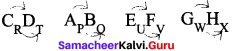We find that when we take 1st & 3rd letter & 2nd & 4th letter as 2 pairs, the 3rd letter is the next letter alphabetically to the 1st letter.
Similarly the 4th letter is alphabetically the next letter of the 2nd letter.
i.e CD, AB, EF, GH & PQ, UV, WX
Only in CRDT, we have T instead of ‘S’. So,
in CRDT ⇒ Option (a)

B. The four groups of letters are
If we notice, we find that 2 letters are missing in the sequence, ie.
HIJKLMNOPQ IJKLMNOPQR
LKLMNOPQRS ABCDEFGHI
We find that only in ADGI, the difference is only one letter between G & I.
Hence it is the odd one out.Question (ii)
A group of letters are given. A numerical code has been given to each letter. These letters have to be unscrambled into a meaningful word. Find out the code for the word so formed from the 4 answers given.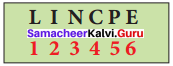(a) 2 3 4 1 5 6
(b) 5 6 3 4 2 1
(c) 6 1 3 5 2 4
(d) 4 2 1 3 5 6
(b) 5 6 3 4 2 1
hint:
Given code isOption (a) is 234156. When we substitute number for each letter from code, we get,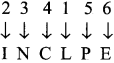Option (b) is 563421, similarly, we get 5 6 3 4 2 1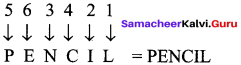Option (c) is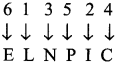Option (d) is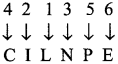So, only in option b, we get a meaningful word, i.e PENCIL.

Question (iii)
In questions I and II, there are based on code language. Find the correct answer from the four alternatives given. A
C In a certain code, ‘M E D I C I N E’ is coded as ‘COMPUTER’ written in the same code?
(a) C N P R V U F Q
(b) C M N Q T U D R
(c) R F U V Q N P C
(d) R N V F T U D Q
(C) R F U V Q N P C
Hint:
It is given that in a certain code M E D I C I N E is coded as E O J D J E F M When we observe the word & the code, we find that, there is a pattern.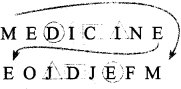[to understand, see the matching shapes]
To get the code from the word, we follow the below steps
1.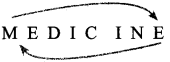swap 1st & last letters, so we get
E [ E D I C I N ] M
2. For the middle letters, replace the letters with their alphabetically next letters, so we get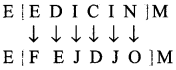3. Now we have to reverse the order of the middle letters in the bracket, so we get
E[O J D J E F]M = E O J D J E F M
Thus we get the code.
So similarly, we have to follow the 3 steps to get code, therefore:
for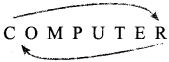Step 1: Swap 1 st & last letters, so we get
R [O M P U T E ]C
Step 2: For the middle letters, replace the letters with alphabetically next letters, so we get
R [P N Q V U F] C
Step 3: Reverse the word of letters in the bracket to we get
R [F U V Q N P] C
∴ Ans is R F U V Q N P C ⇒ Option C

Question (iv)
If the word ‘PHONE’ is coded as ‘S K R Q H’, how will ‘R A D I O’ be coded?
(a) S C G N H
(b) V R G N G
(c) U D G L R
(d) S D H K Q
(C) U D G L R
hint:
If PHONE is coded as S K R Q H
Find that code for R A D I O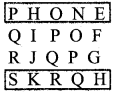We find that we get the code, by 3rd letter alphabetically so, from P, skipping Q & R, we get S.
Similarly, from H, skipping I & J, we get K
Like wise for R A D I O, skipping the 2 alphabets
From R, skip S & T → U
From A, skip B & C → D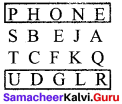From D skip E & F → G
From I skip J & K → L
From O skip P & Q → R
is U D G L R ⇒ Option cQuestion 2.
Fill in the blanks (Use Atbash Cipher that is given in code 3)
For this question, we need to use Atbash cipher. For Atbash cipher, first we write the alphabets from A to Z and then in reverse from Z to A below that.
ABCDEFGHIJKLMNOPQRSTUVWXYZ
ZYXWVUTSRQPONMLKJIHGFEDCBA

Question (i)
G Z N R O = …………
TAMIL
Hint:
Now to solve, we look up the corresponding letter from the table to replace in code to get the actual word.
So, for G Z N R O, from table,
for G, it is T
for Z, It is A
for N, it is M
for R, it is I
for O, it is L
So , the actual word is TAMIL

Question (ii)
V M T O R H S = ………
ENGLISH
Hint:
V M T O R H S
To solve, we look up the corresponding letter from table to replace in code to get the actual word.
For V, it is E
for M, it is N
for T, it is G
for O, it is L
for R, it is I
for H, it is S
for S, it is H
Therefore we get E N G L I S H = ENGLISH

Question (iii)
N Z G S V N Z G R X H = ………
MATHEMATICS
Hint:
Similarly as above for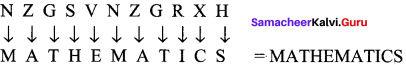Question (v)
H X R V M X V = ………..
SCIENCE
Hint: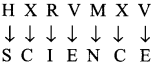Question (v)
HLXRZO HXRVMX V = ………..
SOCIAL SCIENCE
Hint: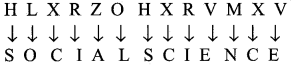Question 3.
Match the following (a = 00 ……… Z = 25)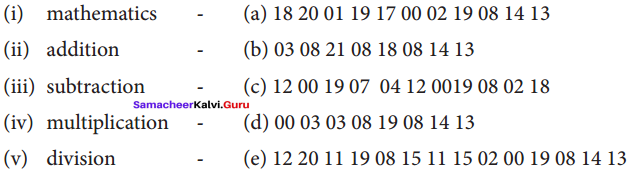(i) – c
(ii) – d
(iii) – a
(iv) – e
(v) – b
Solution:
Given that the code is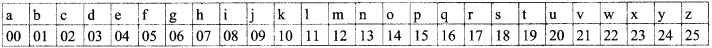Hint:
(i) Mathematics is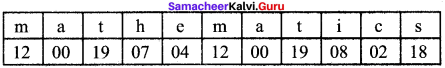So matching option is c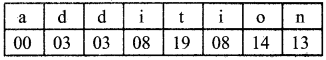Matching option is d

(iii) Subtraction is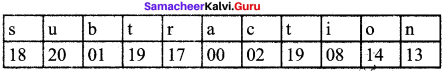Matching option is a

(iv) multiplication is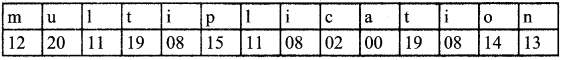Matching option is e, however instead of 25, it should be 08.

(v) division is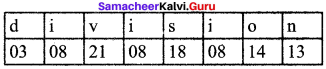Matching option is b

Question 4.
Frame Additive cipher table (key = 4).
Solution:
Step 1 : write all alphabets
Step 2 : Assign numbers to each alphabet starting from 00 till 25.
Step 3 : add key value (here it is 4) to the numbers assigned in step 2 to form cipher table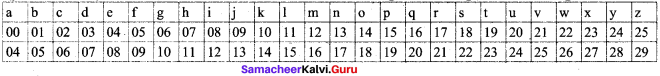Question 5.
A message like “Good Morning” written in reverse would instead be “Doog Gninrom”. In the same way decode the sentence given below: “Ot dnatsrednu taht scitamehtam nac eb decneirepxe erehwreve ni erutan dna laer efil.”
Solution:
Given that good morning written in reverse is doog gninrom.
We have to decode the below by reversing, so,
Ot dnatsrednu taht scitamehtam nac eb decneirepxe erehwreve ni erutan dna laer efil.
to understand that mathematics can be experienced everywhere in nature and real life.Question 6.
Decode the given Pigpen Cipher text and compare your answer to get the Activity 3 result.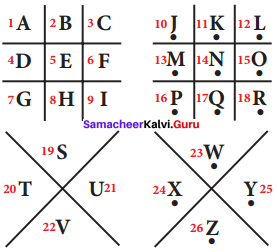(i) The room number in which the treasure took place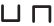(ii) Place of the treasure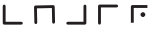(iii) The name of the treasure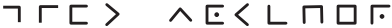Solution:
(i) The room in which the treasure took place = 28
(ii) The place of treasure = Chair
(iii) Identity of treasure = Gift voucher.